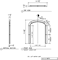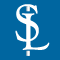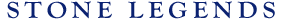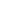HOMEPRODUCTSIDEA CENTERSERVICESTECHNICALABOUT USHello Guest Login  |  Become Member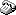800-398-1199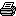214-398-1293MapEmail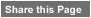Click to Share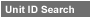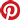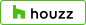Select which variables you would like to search by.Type DescriptionPrimary ViewPrimary ModifierUnit NameView Definitions

View Definitions

View DefinitionsX-Dim Y-Dim Z-Dim XX-Dim YY-Dim ZZ-Dim R-Dim D-Dim P-Dim
 Click column heading to re-sort results list.Product Name Primary View Modifier Pri Dimx  y  z Alt Dimxx  yy  zz Alt2 Dimr  d  p E\$timated Thumbnail Beckman2 Marcus4 SurroundDoor Eyebrow Key X = 57-1/2" Y = 48-1/2"Z = 8-5/16" XX = 79-1/2" YY = 74-1/8"ZZ = 4-5/16" R = 52-1/4" D = n/aP = n/a \$ 999999.00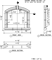Belton1 SurroundDoor SquareTop Key X = 196-1/2" Y = 88-1/2"Z = 5-3/8" XX = 208-1/2" YY = 96-3/4"ZZ = 2-1/4" R = n/a D = n/aP = n/a \$ 999999.00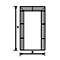Bolt1WedgeThreshold SurroundDoor Eyebrow Threshold X = 36" Y = 105"Z = 9-7/8" XX = 51-1/2" YY = 105"ZZ = 2-3/4" R = 26-5/8" D = n/aP = n/a \$ 999999.00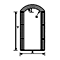Chamber Medallion SurroundDoor SquareTop X = 152" Y = 120"Z = 5-1/8" XX = 168" YY = 133-3/8"ZZ = 3-5/8" R = n/a D = n/aP = n/a \$ 999999.00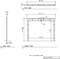Consett1 Orandall1 SurroundDoor SquareTop Pediment X = 81-3/4" Y = 97"Z = 8-1/16" XX = n/a YY = 147-3/16"ZZ = 2-1/4" R = n/a D = n/aP = n/a \$ 999999.00Jamison1 SurroundDoor CircleTop X = 72-1/2" Y = n/aZ = 6-1/8" XX = n/a YY = n/aZZ = n/a R = n/a D = n/aP = n/a \$ 999999.00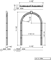Jamison1 Chamfer10C SurroundDoor Eyebrow Key X = 192" Y = 145-7/8"Z = 18" XX = 207" YY = 180-1/8"ZZ = 6-1/8" R = 204" D = n/aP = n/a \$ 999999.00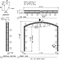Jamison10 Recess12D SurroundDoor CircleTop DividersHorizontal X = 72-1/2" Y = 100-3/4"Z = 7" XX = 88-7/16" YY = 289-3/4"ZZ = 5-9/16" R = 36-1/4" D = n/aP = n/a \$ 999999.00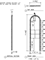Jamison2 Legend Elite SurroundDoor CircleTop X = 36" Y = 72"Z = 5-1/2" XX = n/a YY = n/aZZ = n/a R = 18" D = n/aP = n/a \$ 142.20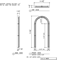Jamison2 Legend Elite SurroundDoor CircleTop X = 60" Y = 72"Z = 5-1/2" XX = n/a YY = n/aZZ = n/a R = 30" D = n/aP = n/a \$ 158.00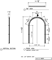Jamison2 Plinths SurroundDoor CircleTop Key X = 81-1/4" Y = 208-1/4"Z = 11" XX = 92-1/2" YY = 248-3/8"ZZ = n/a R = 40-1/8" D = n/aP = n/a \$ 434.37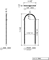Jamison4 Chamfer10D SurroundDoor CircleTop Key X = 44-1/8" Y = 71-1/4"Z = 8" XX = 64-5/8" YY = 107"ZZ = n/a R = 22-1/16" D = n/aP = n/a \$ 999999.00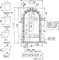Jamison4 Chamfer10D SurroundDoor SquareTop Key X = 120-1/8" Y = 69-1/4"Z = 8" XX = 136" YY = 97-1/8"ZZ = 6-1/2" R = n/a D = n/aP = n/a \$ 999999.00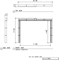Jamison5 Chamfer10H SurroundDoor CircleTop Key X = 24-1/2" Y = 63-1/2"Z = 9" XX = 41-1/2" YY = 85-3/4"ZZ = 4-5/8" R = 12-1/4" D = n/aP = n/a \$ 999999.00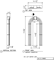Jolie1 SurroundDoor Eyebrow DoubleSide X = 48" Y = 89-1/16"Z = 14" XX = 72" YY = 106-1/4"ZZ = n/a R = 58-1/4" D = n/aP = n/a \$ 999999.00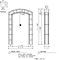Justin17 SurroundDoor Eyebrow Key X = 99" Y = 98"Z = 4" XX = 111" YY = 116-7/8"ZZ = 2-1/2" R = 148" D = n/aP = n/a \$ 999999.00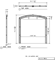Justin2 SurroundDoor Eyebrow Plinth X = 49" Y = 124-1/4"Z = 7-3/4" XX = 75" YY = 145-3/4"ZZ = 7-1/4" R = 37-7/8" D = n/aP = n/a \$ 999999.00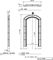Marcus3 Legend Elite SurroundDoor Eyebrow X = 72" Y = 72"Z = 5-1/2" XX = n/a YY = n/aZZ = n/a R = 45" D = n/aP = n/a \$ 166.32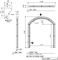Marcus4A SurroundDoor EllipticalTop X = 75-1/8" Y = 111"Z = 5-3/4" XX = 91-1/8" YY = n/aZZ = n/a R = 56-3/4" D = n/aP = n/a \$ 999999.00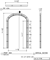Marshall1 SurroundDoor Eyebrow X = 63-3/4" Y = 116-3/16"Z = 8" XX = n/a YY = n/aZZ = n/a R = 66-1/8" D = n/aP = n/a \$ 999999.00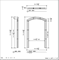Marshall1 Legend Elite SurroundDoor SquareTop X = 48" Y = 72"Z = 5-1/2" XX = n/a YY = n/aZZ = n/a R = n/a D = n/aP = n/a \$ 190.08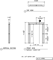Marshall1 Marshall2 SurroundDoor Eyebrow Key X = 108" Y = 85-3/8"Z = 7-1/4" XX = 124" YY = 108"ZZ = n/a R = 142-7/16" D = n/aP = n/a \$ 999999.00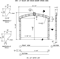Marshall12 SurroundDoor Eyebrow Plinth X = 76-1/2" Y = 102"Z = 9" XX = 84-1/4" YY = 121-13/16"ZZ = 8" R = 79" D = n/aP = n/a \$ 999999.00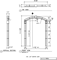Marshall1A Legend Elite SurroundDoor CircleTop X = 48" Y = 72"Z = 7" XX = n/a YY = n/aZZ = n/a R = 24" D = n/aP = n/a \$ 266.00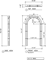Marshall2 SurroundDoor Eyebrow X = 47-1/2" Y = 100-3/16"Z = 5-1/2" XX = 59-1/2" YY = 106-3/16"ZZ = 1-3/4" R = 46" D = n/aP = n/a \$ 999999.00Marshall6 SurroundDoor Eyebrow Ear X = 191" Y = 94"Z = 3-1/2" XX = 207" YY = 102"ZZ = n/a R = n/a D = n/aP = n/a \$ 999999.00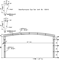Marshall6 SurroundDoor Eyebrow Ear X = 118-1/2" Y = 95-1/4"Z = 3-1/2" XX = 134-1/2" YY = 103-1/4"ZZ = n/a R = n/a D = n/aP = n/a \$ 999999.00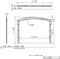Marshall6 Beckman SurroundDoor CircleTop Ear X = 54" Y = 107"Z = 3-1/2" XX = 70" YY = 115"ZZ = n/a R = n/a D = n/aP = n/a \$ 999999.00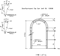Odyssey1A SurroundDoor SquareTop Plinth X = 75" Y = 96"Z = 5" XX = 91" YY = 104"ZZ = n/a R = n/a D = n/aP = n/a \$ 999999.00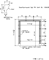Odyssey6 SurroundDoor CircleTop X = 111-1/2" Y = 157-1/2"Z = 8" XX = 131-1/2" YY = 223-1/4"ZZ = n/a R = 55-3/4" D = n/aP = n/a \$ 999999.00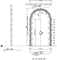Salem1 Eyebrow SurroundDoor Eyebrow Key X = 72-1/2" Y = 129-11/16"Z = 6-1/2" XX = 86-1/2" YY = 141-11/16"ZZ = 4-1/2" R = n/a D = n/aP = n/a \$ 999999.00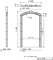Salem2 SurroundDoor Eyebrow Ear X = 54" Y = 101-1/2"Z = 6-1/2" XX = 66" YY = 118-3/8"ZZ = 4-1/2" R = 41-1/8" D = n/aP = n/a \$ 999999.00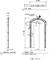Salem2 Legend Elite SurroundDoor Eyebrow X = 48" Y = 72"Z = 6-1/2" XX = n/a YY = n/aZZ = n/a R = 30" D = n/aP = n/a \$ 168.48Salem2 Legend Elite SurroundDoor Eyebrow X = 72" Y = 72"Z = 6-1/2" XX = n/a YY = n/aZZ = n/a R = 45" D = n/aP = n/a \$ 196.56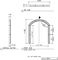Salem2 Legend Elite SurroundDoor Eyebrow X = 60" Y = 72"Z = 6-1/2" XX = n/a YY = n/aZZ = n/a R = 37-1/2" D = n/aP = n/a \$ 187.20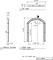Salem2 Legend Elite SurroundDoor Eyebrow Ear X = 72" Y = 74"Z = 6-1/2" XX = 84" YY = 90-3/8"ZZ = 4-1/2" R = 72" D = n/aP = n/a \$ 999999.00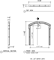Salem3 SurroundDoor SquareTop X = 108" Y = 96"Z = 6-7/16" XX = 124" YY = 104"ZZ = 4" R = n/a D = n/aP = n/a \$ 999999.00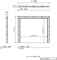Vincent1 SurroundDoor SquareTop Key X = 74-15/16" Y = 108"Z = 5" XX = n/a YY = 115-3/4"ZZ = 4-7/8" R = n/a D = n/aP = n/a \$ 999999.00Vincent1 Legend Elite SurroundDoor EllipticalTop X = 60" Y = 72"Z = 4-7/8" XX = n/a YY = n/aZZ = n/a R = 10-3/8" D = n/aP = n/a \$ 181.20